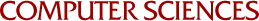On Multivariate Polynomial Interpolation

Nira Dyn and Amos Ron
1989

A class of spaces of multivariate polynomials, closed under differentiation, is studied and corresponding classes of well posed Hermite-type interpolation problems are presented. All Hermite-type problems are limits of well posed LaGrange problems. The results are based on a duality between certain spaces of multivariate exponential-polynomials H and corresponding spaces of multivariate polynomials P, used by Dyn and Ron (1988) to establish the approximation order of the span of translates of exponential box splines. In the interpolation theory P is the space of interpolating polynomials and H characterizes the interpolation points and the interpolation conditions, both spaces being defined in terms of a set of hyperplanes in IR. This geometric approach extends the work of Chung and Yao (1977) on Lagrange interpolation, and also a subset of the Hermite-type problems considered via the Newton scheme, by several authors (see Gasca and Maetzu (1982) and references therein). For a different approach to the interpolation problem see Chui and Lai (1988). It is the systematic and unified analysis of a wide class of interpolation problems which is the main contribution of this paper to the study of multivariate polynomial interpolation.